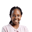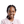Trusted answers to developer questions
Trusted Answers to Developer Questions

Related Tags

scipy
linalg
python
communitycreator

# What is the scipy.linalg.coshm() function in Python?Joy Kareko

Grokking Modern System Design Interview for Engineers & Managers

Ace your System Design Interview and take your career to the next level. Learn to handle the design of applications like Netflix, Quora, Facebook, Uber, and many more in a 45-min interview. Learn the RESHADED framework for architecting web-scale applications by determining requirements, constraints, and assumptions before diving into a step-by-step design process.

### Overview

Linear Algebra is a branch of mathematics that contains functions and equations useful in mathematical computations. Python’s scipy library is used for mathematical and scientific calculations. It includes the module linalg that contains linear algebra functions.

The coshm() function returns the hyperbolic cosine of a matrix.

### Syntax

scipy.linalg.coshm(A)


### Parameters

This function takes the parameter, A, which represents the input matrix.

### Return value

This function returns another matrix containing the hyperbolic cosine of A.

### Example

import numpy as npimport scipyfrom scipy.linalg import coshmA = np.matrix([[0.234,0.675],[3.456,0.987]])print(A)print('--------------------------')B = coshm(A)print(B)
Hit run to see the results! Try changing input arguments and observe the results.

### Explanation

• Lines 1–2: We import the libraries, numpy and scipy.
• Line 3: We import the linalg module from scipy.
• Line 5–6: We create a matrix, A, and print it.
• Line 9: We calculate the hyperbolic cosine for the matrix A, and store it in matrix B.
• Line 10: We print B.

RELATED TAGS

scipy
linalg
python
communitycreator

CONTRIBUTORJoy Kareko

Grokking Modern System Design Interview for Engineers & Managers

Ace your System Design Interview and take your career to the next level. Learn to handle the design of applications like Netflix, Quora, Facebook, Uber, and many more in a 45-min interview. Learn the RESHADED framework for architecting web-scale applications by determining requirements, constraints, and assumptions before diving into a step-by-step design process.

Keep Exploring

Learn in-demand tech skills in half the time